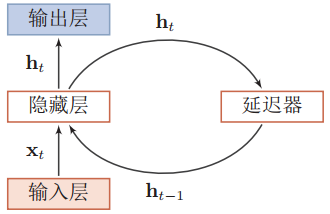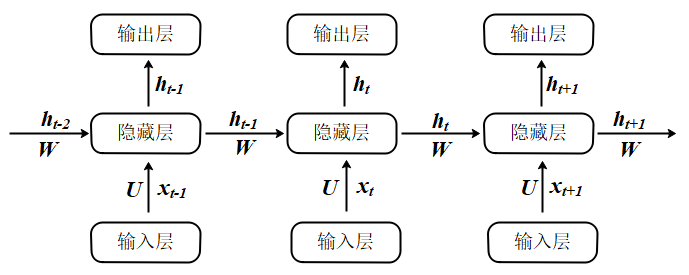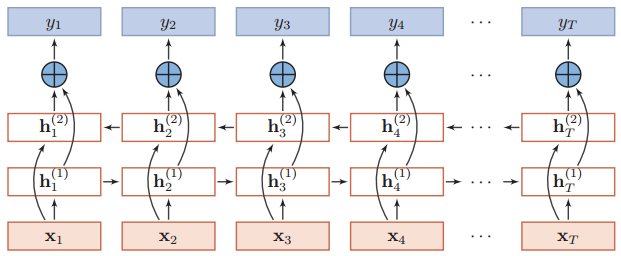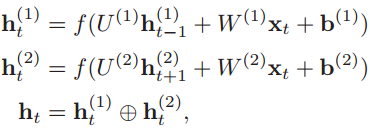# 2 循环神经网络¶

## 2.2 单向循环神经网络结构¶$$h_t=f(U \times x_t + w \times h_{t-1} + b) \tag{1}$$

$$h_{t-1}=f(U \times x_{t-1} + w \times h_{t-2} + b) \tag{2}$$ 将（1）式和（2）式结合，也就有： $$h_t=f(U \times x_t + w \times f(U \times x_{t-1} + w \times h_{t-2} + b) + b) \tag{3}$$ 通过这种方式，第$t$时刻的输出与之前时刻的输出关联起来，也就有了之前的“记忆”。

## 2.3 双向循环网络结构¶# 3 TensorFlow2实现循环神经网络¶

## 3.1 单层节点¶

In :
import tensorflow as tf


In :
x = tf.random.normal([4, 80, 100])


In :
cell = tf.keras.layers.SimpleRNNCell(64)  # 64的意思是经过神经元后，维度转化为64维，[b, 100] -> [b, 64]，b是句子数量


In :
ht0 = tf.zeros([4, 64])  # 因为

In :
xt0 = x[:, 0, :]

In :
out, ht1 = cell(xt0, [h0])


out是传递给下一层的值，ht1经过一次神经元后的转台。我们看看这两者的状态：

In :
out.shape, ht1.shape

Out:
(TensorShape([4, 64]), TensorShape([4, 64]))

In :
id(out), id(ht1)

Out:
(140525368605520, 140525368605520)

## 3.2 多层节点¶

In :
x = tf.random.normal([4, 80, 100])
xt0 = x[:, 0, :]


In :
# 第一层节点
cell = tf.keras.layers.SimpleRNNCell(64)
# 第二层节点
cell2 = tf.keras.layers.SimpleRNNCell(64)


In :
state0 = [tf.zeros([4, 64])]
state1 = [tf.zeros([4, 64])]


In :
out0, state0 = cell(xt0, state0)
out1, state1 = cell2(out, state1)


In [ ]:
for word in tf.unstack(x, axis=1):
out0, state0 = cell(xt0, state0)
out1, state1 = cell2(out, state1)


## 3.3 SimpleRNNCell实现完整RNN实现文本分类¶

In :
import os
import tensorflow as tf
import numpy as np
from tensorflow import keras
from tensorflow.keras import layers

In :
tf.random.set_seed(22)
np.random.seed(22)
os.environ['TF_CPP_MIN_LOG_LEVEL'] = '2'


In :
total_words = 10000  # 常见词汇数量
batchsz = 128
embedding_len = 100
(x_train, y_train), (x_test, y_test) = keras.datasets.imdb.load_data(num_words=total_words)

In :
max_review_len = 80  # 每个句子最大长度

In :
db_train = tf.data.Dataset.from_tensor_slices((x_train, y_train))
db_train = db_train.shuffle(1000).batch(batchsz, drop_remainder=True)  # drop_remainder是指最后一个batch不足128个则丢弃
db_test = tf.data.Dataset.from_tensor_slices((x_test, y_test))
db_test = db_test.batch(batchsz, drop_remainder=True)

In :
print('x_train shape:', x_train.shape, tf.reduce_max(y_train), tf.reduce_min(y_train))
print('x_test shape:', x_test.shape)


x_train shape: (25000, 80) tf.Tensor(1, shape=(), dtype=int64) tf.Tensor(0, shape=(), dtype=int64)
x_test shape: (25000, 80)


In :
class RNN(keras.Model):
def __init__(self, units):
super(RNN, self).__init__()
# 创建初始状态矩阵
self.state0 = [tf.zeros([batchsz, units])]
self.state1 = [tf.zeros([batchsz, units])]
# 对文本数据进行转换为矩阵
# 每句80个单词，每个单词100维矩阵表示  [b, 80]  --> [b, 80, 100]
self.embedding = layers.Embedding(total_words, embedding_len, input_length=max_review_len)
# 循环网络层, 语义提取
# [b, 80, 100]--> [b, 64]
self.rnn_cell0 = layers.SimpleRNNCell(units, dropout=0.2)  # 第一层rnn
self.rnn_cell1 = layers.SimpleRNNCell(units, dropout=0.2)  # 第二层rnn
# 全连接层
# [b, 64] --> [b, 1]
self.outlayer = layers.Dense(1)

def call(self, inputs, training=None):
# [b, 80]
x = inputs
# embedding: [b, 80] -->[b, 80, 100]
x = self.embedding(x)
# rnn cell:  [b, 80, 100] --> [b, 64]
state0 = self.state0
state1 = self.state1
for word in tf.unstack(x, axis=1): # 在第二个维度展开，遍历句子的每一个单词
#
out0, state0 = self.rnn_cell0(word, state0, training)
out1, state1 = self.rnn_cell1(out0, state1, training)
# out: [b, 64]
x = self.outlayer(out1)
prob = tf.sigmoid(x)
return prob

In :
units = 64
epochs = 4
model = RNN(units)
loss=tf.losses.BinaryCrossentropy(),
metrics=['accuracy'],
experimental_run_tf_function = False
)
model.fit(db_train, epochs=epochs, validation_data=db_test)


Epoch 1/4
193/195 [============================>.] - ETA: 0s - loss: 0.5973 - accuracy: 0.5506Epoch 1/4
195/195 [==============================] - 3s 15ms/step - loss: 0.4002 - accuracy: 0.8220
195/195 [==============================] - 13s 65ms/step - loss: 0.5958 - accuracy: 0.5520 - val_loss: 0.4002 - val_accuracy: 0.8220
Epoch 2/4
193/195 [============================>.] - ETA: 0s - loss: 0.3326 - accuracy: 0.8490Epoch 1/4
195/195 [==============================] - 1s 7ms/step - loss: 0.4065 - accuracy: 0.8224
195/195 [==============================] - 5s 28ms/step - loss: 0.3315 - accuracy: 0.8492 - val_loss: 0.4065 - val_accuracy: 0.8224
Epoch 3/4
193/195 [============================>.] - ETA: 0s - loss: 0.1839 - accuracy: 0.9180Epoch 1/4
195/195 [==============================] - 1s 7ms/step - loss: 0.5103 - accuracy: 0.8193
195/195 [==============================] - 6s 28ms/step - loss: 0.1841 - accuracy: 0.9182 - val_loss: 0.5103 - val_accuracy: 0.8193
Epoch 4/4
193/195 [============================>.] - ETA: 0s - loss: 0.0960 - accuracy: 0.9574Epoch 1/4
195/195 [==============================] - 1s 7ms/step - loss: 0.6747 - accuracy: 0.8083
195/195 [==============================] - 5s 28ms/step - loss: 0.0958 - accuracy: 0.9575 - val_loss: 0.6747 - val_accuracy: 0.8083

Out:
<tensorflow.python.keras.callbacks.History at 0x7fce3bc7d390>

## 3.4 高层API实现RNN¶

In :
model = keras.Sequential([
layers.Embedding(total_words, embedding_len, input_length=max_review_len),
layers.SimpleRNN(units, dropout=0.2, return_sequences=True, unroll=True),
layers.SimpleRNN(units, dropout=0.2,  unroll=True),
layers.Dense(1, activation='sigmoid')
])
metrics=['accuracy'])
history1 = model.fit(db_train, epochs=epochs, validation_data=db_test)
loss, acc = model.evaluate(db_test)
print('准确率:', acc) # 0.81039


Train for 195 steps, validate for 195 steps
Epoch 1/4
195/195 [==============================] - 11s 54ms/step - loss: 0.6243 - accuracy: 0.6187 - val_loss: 0.4674 - val_accuracy: 0.7830
Epoch 2/4
195/195 [==============================] - 6s 29ms/step - loss: 0.3770 - accuracy: 0.8352 - val_loss: 0.4061 - val_accuracy: 0.8230
Epoch 3/4
195/195 [==============================] - 6s 29ms/step - loss: 0.2527 - accuracy: 0.9007 - val_loss: 0.4337 - val_accuracy: 0.8201
Epoch 4/4
195/195 [==============================] - 6s 29ms/step - loss: 0.1383 - accuracy: 0.9493 - val_loss: 0.5847 - val_accuracy: 0.8076
195/195 [==============================] - 1s 7ms/step - loss: 0.5847 - accuracy: 0.8076



posted @ 2020-07-14 07:38  奥辰  阅读(183)  评论(0编辑  收藏